# Lattice

(diff) ← Older revision | Latest revision (diff) | Newer revision → (diff)

structure

A partially ordered set in which each two-element subset has both a least upper and a greatest lower bound. This implies the existence of such bounds for every non-empty finite subset.

## Contents

### Examples.

1) A linearly ordered set (or chain)where for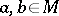, if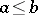, then2) The subspaces of a vector space ordered by inclusion, where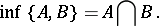3) The subsets of a given set ordered by inclusion, where4) The non-negative integers ordered by divisibility:iffor a certain; where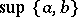is the least common multiple ofand, and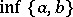is the greatest common divisor ofand.

5) The real-valued functions defined on the interval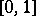and ordered by the condition:iffor all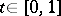, wherein which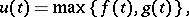and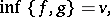in which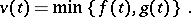Letbe a lattice.becomes a universal algebra with two binary operations if one defines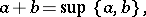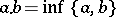(the symbols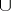andorand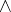are often used instead ofand). This universal algebra satisfies the following identities: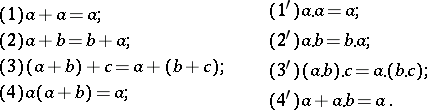Conversely, ifis a set with two binary operations that have the properties –, (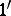)–(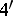) mentioned above, then an ordercan be imposed onby settingif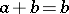(it turns out in this case thatif and only if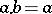). The resulting partially ordered set will be a lattice in whichIn this way a lattice can be defined as a universal algebra satisfying the identities –, ()–(), i.e. lattices form a variety of universal algebras.

If a partially ordered set is regarded as a small category, then it is a lattice if and only if has products and coproducts of pairs of objects.

Ifand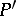are lattices and if:is an isomorphism of partially ordered sets, thenis also an isomorphism of the corresponding universal algebras, i.e.for any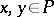. However, an arbitrary isotone mapping ofintois not necessarily a homomorphism of these lattices considered as universal algebras. Thus, for any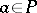, the mappings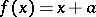and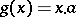are isotone mappings of the latticeinto itself, but they are homomorphisms if and only ifis a distributive lattice. However, the first of these mappings is a homomorphism of the semi-latticewith the operation, and the second is a homomorphism of the semi-latticewith the operation. The class of all lattices forms a category if homomorphisms are taken as morphisms.

An anti-homomorphism of a latticeinto a latticeis a mapping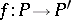such thatfor any. A composite of two anti-homomorphisms is a homomorphism. A partially ordered set that is anti-isomorphic to a lattice is a lattice.

By coordinatization of a lattice is meant the finding of an algebraic system (most often a universal algebra) such that the given lattice is isomorphic to the lattice of subsystems, to the lattice of congruences or to some other lattice associated with this algebraic system or universal algebra. An arbitrary lattice with a 0 and a 1 is coordinatized by the partially ordered semi-group of its residual mappings (cf. Residual mapping) into itself, and turns out to be isomorphic to the lattice of right annihilators of this semi-group. The semi-group itself is a Baer semi-group, i.e. the right and left annihilators of each of its elements are generated by idempotents.

The most important results are obtained for lattices subjected to some kind of additional restrictions (see Algebraic lattice; Atomic lattice; Brouwer lattice; Vector lattice; Modular lattice; Distributive lattice; Multiplicative lattice; Orthomodular lattice; Complete lattice; Continuous lattice; Free lattice; Lattice with complements; Boolean algebra). For specific problems in the theory of lattices see Ideal; Filter; Completion, MacNeille (of a partially ordered set). Algebraic systems that are at the same time lattices play a special role (see Lattice-ordered group). The majority of applications of the theory of lattices are associated with Boolean algebras. Other classes of lattices have been used in quantum mechanics and physics.

The concept of a lattice first arose in the late 19th century and was connected with the fact that many results about the set of ideals of a ring or the set of normal subgroups of a group seemed analogous and could be proved in the framework of modular lattices. As an independent branch of algebra, the theory of lattices was developed in the 1930s.

How to Cite This Entry:
Lattice. Encyclopedia of Mathematics. URL: http://encyclopediaofmath.org/index.php?title=Lattice&oldid=11553
This article was adapted from an original article by L.A. Skornyakov (originator), which appeared in Encyclopedia of Mathematics - ISBN 1402006098. See original article